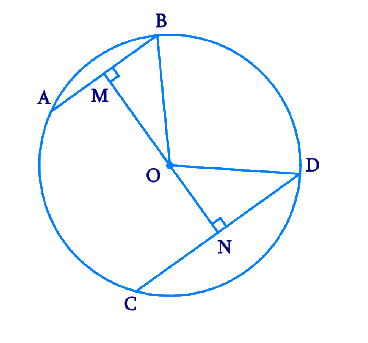# Ex.10.6 Q2 Circles Solution - NCERT Maths Class 9

Go back to  'Ex.10.6'

## Question

Two chords \begin {align}{AB}\end {align} and \begin {align}{CD}\end {align} of lengths \begin {align}\text{5 cm}\end {align} and \begin {align}\text{11 cm}\end {align} respectively of a circle are parallel to each other and are on opposite sides of its centre. If the distance between \begin {align}{AB}\end {align} and \begin {align}{CD}\end {align} is \begin {align}\text{6 cm,}\end {align} find the radius of the circle.

Video Solution
Circles
Ex 10.6 | Question 2

## Text Solution

What is known?

Two parallel chords of lengths $$5\rm{ cm}$$ and $$11\rm{ cm}$$ and the distance between parallel chords is $$6\rm{ cm.}$$

#### What is unknown?

Reasoning:

The perpendicular drawn from the centre of the circle to the chords, bisects it.

Pythagoras theorem states that

(Side $$1)^{2}$$ $$+$$ (Side $$2)^{2} =$$ (Hypotenuse )$$^{2}$$

Steps:

Draw $$2$$ parallel chords \begin {align}{AB}\end {align} and \begin {align}{CD}\end {align} of lengths \begin {align}\text{5 cm}\end {align} and \begin {align}\text{11 cm.}\end {align} Let the centre of the circle be \begin {align}{O. }\end {align} Join one end of each chord to the centre. Draw $$2$$ perpendiculars \begin {align}{OM}\end {align} and \begin {align}{ON}\end {align} to \begin {align}{AB}\end {align} and \begin {align}{CD }\end {align} respectively which bisects the chords.\begin {align}{AB}&=5 \mathrm{cm} \\ {CD}&=11 \mathrm{cm} \\ {MB}&=2.5 \mathrm{cm} \\ {ND}&=5.5 \mathrm{cm}\end{align}

Let  $$OM = x \,\,\rm{cm}$$

and  $$ON = 6 – x\,\,\rm{cm}$$

Consider \begin{align}\Delta {OMB}\end {align}

By Pythagoras theorem,

\begin{align} {OM}^{2}+{MB}^{2} &={OB}^{2} \\ x^{2}+2.5^{2} &={OB}^{2} \\ x^{2}+6.25 &={OB}^{2} \ldots .(1) \end{align}

Consider \begin{align}\Delta {OND}\end {align}

By Pythagoras theorem,

\begin{align}{ON}^{2}+{ND}^{2}&={OD}^{2} \\ (6-x)^{2}+5.5^{2}&={OD}^{2} \\ \begin{Bmatrix} 36+x^{2} \\ -12 x+30.25 \end{Bmatrix} &={OD}^{2} \\ \begin{Bmatrix} x^{2}-12 x \\ +66.25 \end{Bmatrix} &={OD}^{2} \ldots .(2)\end{align}

\begin{align} {OB}\end{align} and \begin{align} {OD}\end{align} are the radii of the circle. Therefore \begin{align} {OB = OD.}\end{align}

Equating ($$1$$) and ($$2$$) we get,

\begin{align}x^{2}+6.25&=x^{2}-12 x+66.25 \\ 12 x&=60 \\ x&=5\end{align}

Substituting the value of \begin{align} x\end{align} in ($$1$$) or ($$2$$) we get the radius of circle $$= 5.59 \rm cm.$$

Video Solution
Circles
Ex 10.6 | Question 2

Learn from the best math teachers and top your exams

• Live one on one classroom and doubt clearing
• Practice worksheets in and after class for conceptual clarity
• Personalized curriculum to keep up with school